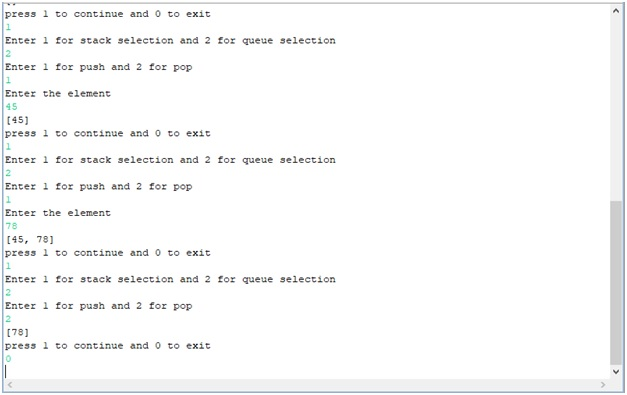# Using queue and stack in a single Java program using loops

In this article, we will be writing a simple java program to use stack and queue in a single program to understand the difference between the two.
Submitted by Raunak Goswami, on October 17, 2018

As you are aware that stack follows LIFO principle i.e first in first out and queue follows FIFO principle i.e last in first out, below is the simple java code which better illustrates the difference between the two.

```import java.util.LinkedList;
import java.util.Queue;
import java.util.Scanner;
import java.util.Stack;

public class stackqueue {
public static void main(String[] args) {
int k=1;
Stack<Integer> stack=new Stack<Integer>();
Scanner sc =new Scanner(System.in);
do
{
int j=0,i,ent;
System.out.println("Enter 1 for stack selection and 2 for queue selection");
j=sc.nextInt();
if(j==1)
{
System.out.println("Enter 1 for push and 2 for pop");
i=sc.nextInt();
if(i==1)
{
System.out.println("Enter the element");
ent =sc.nextInt();
stack.push(ent);
System.out.println(stack);
}
else
{
stack.pop();
System.out.println(stack);
}
}
else
{
System.out.println("Enter 1 for push and 2 for pop");
i=sc.nextInt();
if(i==1)
{
System.out.println("Enter the element");
ent =sc.nextInt();
System.out.println(queue);
}
else
{
queue.remove();
System.out.println(queue);
}
}
System.out.println("press 1 to continue and 0 to exit");
k=sc.nextInt();
}while(k==1);
}
}
```

OutputTop MCQs